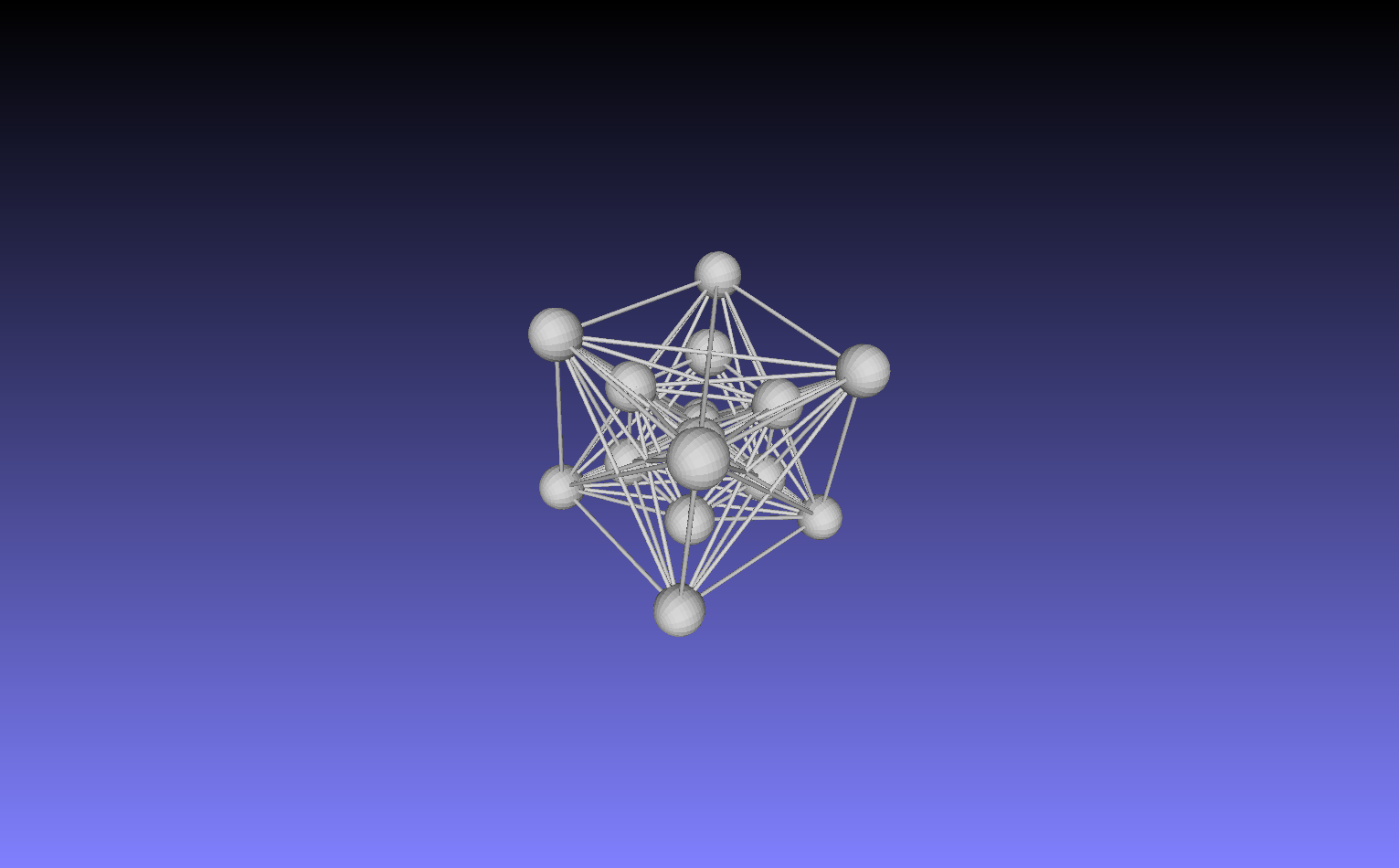# Metatron’s Cube

I was recently asked to create a 3D laser etched crystal of Metatron’s Cube.

The 13 Metatron rings are (or at least can be) taken from the 16 vertices of the tesseract (a 4D geometric figure called an 8-cell) which can be visualized by projecting 4D into 3D as two concentric 3D cubes.

Three of the 16 rings are hidden behind the ring in the front center when projecting the two concentric 3D cubes to 2D (or looking at it from just the correct angle on a corner and ignoring the perspective error introduced by parallax).

There are many more lines in the Metatron cube than from the typical 32 edges of the 8-cell tesseract connecting the vertices. These come from what is called the “complete graph” of 120 edges from the 16 vertices of the 8-cell. The Platonic solids are all contained (or related by duality) within the 3D projection of this complete graph of the 8-cell with large vertex spheres.

Slightly off angle (notice the 3 hidden spheres are now visible):